Shown in the figure is a circular loop of radius r and resistance R. A variable magnetic field of induction B = B0et is established inside the coil. If the key (K) is closed, the electrical power developed right after closing the switch is equal to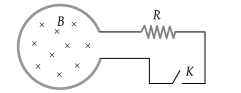(1) $\frac{{B}_{0}^{2}\pi {r}^{2}}{R}$

(2) $\frac{{B}_{0}10{r}^{3}}{R}$

(3) $\frac{{B}_{0}^{2}{\pi }^{2}{r}^{4}R}{5}$

(4) $\frac{{B}_{0}^{2}{\pi }^{2}{r}^{4}}{R}$

Concept Questions :-

Faraday 's law and lenz law
High Yielding Test Series + Question Bank - NEET 2020

Difficulty Level:

A highly conducting ring of radius R is perpendicular to and concentric with the axis of a long solenoid as shown in fig. The ring has a narrow gap of width d in its circumference. The solenoid has a cross-sectional area A and a uniform internal field of magnitude B0. Now beginning at t = 0, the solenoid current is steadily increased so that the field magnitude at any time t is given by B(t) = B0 + αt where α > 0. Assuming that no charge can flow across the gap, the end of the ring which has an excess of positive charge and the magnitude of induced e.m.f. in the ring are respectively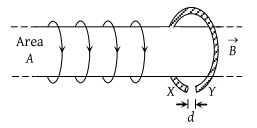(1) X,

(2) X πR2α

(3) Y, πA2α

(4) Y, πR2α

Concept Questions :-

Faraday 's law and lenz law
High Yielding Test Series + Question Bank - NEET 2020

Difficulty Level:

A rectangular loop with a sliding connector of length l = 1.0 m is situated in a uniform magnetic field B = 2T perpendicular to the plane of the loop. Resistance of connector is r = 2Ω. Two resistance of 6Ω and 3Ω are connected as shown in the figure. The external force required to keep the connector moving with a constant velocity v = 2m/s is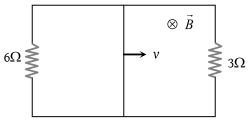(1) 6 N

(2) 4 N

(3) 2 N

(4) 1 N

Concept Questions :-

Motional emf
High Yielding Test Series + Question Bank - NEET 2020

Difficulty Level:

A wire cd of length l and mass m is sliding without friction on conducting rails ax and by as shown. The vertical rails are connected to each other with a resistance R between a and b. A uniform magnetic field B is applied perpendicular to the plane abcd such that cd moves with a constant velocity of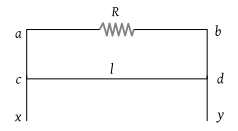(1) $\frac{mgR}{Bl}$

(2) $\frac{mgR}{{B}^{2}{l}^{2}}$

(3) $\frac{mgR}{{B}^{3}{l}^{3}}$

(4) $\frac{mgR}{{B}^{2}l}$

Concept Questions :-

Motional emf
High Yielding Test Series + Question Bank - NEET 2020

Difficulty Level:

A conducting rod AC of length 4l is rotated about a point O in a uniform magnetic field $\stackrel{\to }{B}$ directed into the paper. AO = l and OC = 3l. Then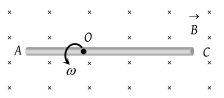(1) ${V}_{A}-{V}_{O}=\frac{B\omega {l}^{2}}{2}$

(2) ${V}_{O}-{V}_{C}=\frac{7}{2}B\omega {l}^{2}$

(3) ${V}_{A}-{V}_{C}=4B\omega {l}^{2}$

(4) ${V}_{C}-{V}_{O}=\frac{9}{2}B\omega {l}^{2}$

Concept Questions :-

Motional emf
High Yielding Test Series + Question Bank - NEET 2020

Difficulty Level:

The figure shows three circuits with identical batteries, inductors, and resistors. Rank the circuits according to the current through the battery (i) just after the switch is closed and (ii) a long time later, greatest first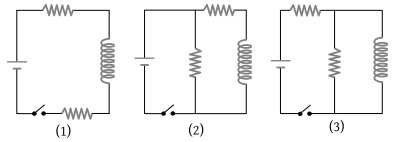(1) (i) i2 > i3 > i1 (i1 = 0) (ii) i2 > i3 > i1

(2) (i) ${i}_{2}<{i}_{3}<{i}_{1}\text{\hspace{0.17em}\hspace{0.17em}}\left({i}_{1}\ne 0\right)$ (ii) i2 > i3 > i1

(3) (i) i2 = i3 = i1 (i1 = 0) (ii) i2 < i3 < i1

(4) (i) ${i}_{2}={i}_{3}>{i}_{1}\text{\hspace{0.17em}\hspace{0.17em}}\left({i}_{1}\ne 0\right)$ (ii) i2 > i3 > i1

Concept Questions :-

LR circuit
High Yielding Test Series + Question Bank - NEET 2020

Difficulty Level:

The network shown in the figure is a part of a complete circuit. If at a certain instant the current i is 5 A and is decreasing at the rate of 103 A/s then VB – VA is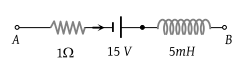(1) 5 V

(2) 10 V

(3) 15 V

(4) 20 V

Concept Questions :-

LR circuit
High Yielding Test Series + Question Bank - NEET 2020

Difficulty Level:

A simple pendulum with bob of mass m and conducting wire of length L swings under gravity through an angle 2θ. The earth’s magnetic field component in the direction perpendicular to swing is B. Maximum potential difference induced across the pendulum is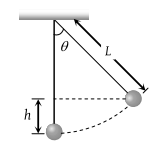(1) $2BL\mathrm{sin}\left(\frac{\theta }{2}\right){\left(gL\right)}^{1/2}$

(2) $BL\mathrm{sin}\left(\frac{\theta }{2}\right)\left(gL\right)$

(3) $BL\mathrm{sin}\left(\frac{\theta }{2}\right){\left(gL\right)}^{3/2}$

(4) $BL\mathrm{sin}\left(\frac{\theta }{2}\right){\left(gL\right)}^{2}$

High Yielding Test Series + Question Bank - NEET 2020

Difficulty Level:

The variation of induced emf (E) with time (t) in a coil if a short bar magnet is moved along its axis with a constant velocity is best represented as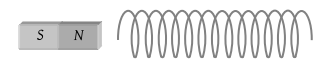(1)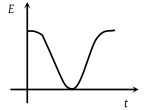(2)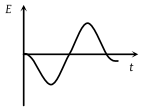(3)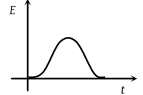(4)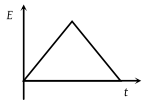Concept Questions :-

Faraday 's law and lenz law
High Yielding Test Series + Question Bank - NEET 2020

Difficulty Level:

A horizontal loop abcd is moved across the pole pieces of a magnet as shown in fig. with a constant speed v. When the edge ab of the loop enters the pole pieces at time t = 0 sec. Which one of the following graphs represents correctly the induced emf in the coil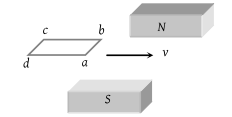(1)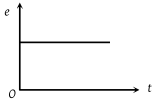(2)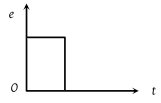(3)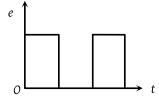(4)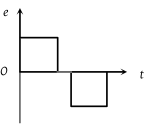Concept Questions :-

Faraday 's law and lenz law